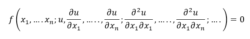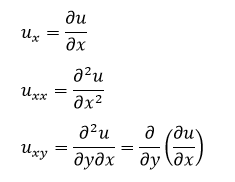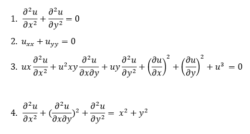# Partial Differential Equation

In Mathematics, a partial differential equation is one of the types of differential equations, in which the equation contains unknown multi variables with their partial derivatives. It is a special case of an ordinary differential equation. In this article, we are going to discuss what is a partial differential equation, how to represent it, its classification and types with more examples and solved problems.

## Partial Differential Equation Definition

A Partial Differential Equation commonly denoted as PDE is a differential equation containing partial derivatives of the dependent variable (one or more) with more than one independent variable. A PDE for a function u(x1,……xn) is an equation of the formThe PDE is said to be linear if f is a linear function of u and its derivatives. The simple PDE is given by;

∂u/∂x (x,y) = 0

The above relation implies that the function u(x,y) is independent of x which is the reduced form of partial differential equation formula stated above. The order of PDE is the order of the highest derivative term of the equation.

## How to Represent Partial Differential Equation?

In PDEs, we denote the partial derivatives using subscripts, such as;In some cases, like in Physics when we learn about wave equations or sound equation, partial derivative, ∂ is also represented by ∇(del or nabla).

## Partial Differential Equation Classification

Each type of PDE has certain functionalities that help to determine whether a particular finite element approach is appropriate to the problem being described by the PDE. The solution depends on the equation and several variables contain partial derivatives with respect to the variables. There are three-types of second-order PDEs in mechanics. They are

• Elliptic PDE
• Parabolic PDE
• Hyperbolic PDE

Consider the example, auxx+buyy+cuyy=0, u=u(x,y). For a given point (x,y), the equation is said to be Elliptic if b2-ac<0 which are used to describe the equations of elasticity without inertial terms. Hyperbolic PDEs describe the phenomena of wave propagation if it satisfies the condition b2-ac>0. For parabolic PDEs, it should satisfy the condition b2-ac=0. The heat conduction equation is an example of a parabolic PDE.

## Partial Differential Equation Types

The different types of partial differential equations are:

• First-order Partial Differential Equation
• Linear Partial Differential Equation
• Quasi-Linear Partial Differential Equation
• Homogeneous Partial Differential Equation

Let us discuss these types of PDEs here.

First-Order Partial Differential Equation

In Maths, when we speak about the first-order partial differential equation, then the equation has only the first derivative of the unknown function having ‘m’ variables. It is expressed in the form of;

F(x1,…,xm, u,ux1,….,uxm)=0

Linear Partial Differential Equation

If the dependent variable and all its partial derivatives occur linearly in any PDE then such an equation is called linear PDE otherwise a nonlinear PDE. In the above example (1) and (2) are said to be linear equations whereas example (3) and (4) are said to be non-linear equations.

Quasi-Linear Partial Differential Equation

A PDE is said to be quasi-linear if all the terms with the highest order derivatives of dependent variables occur linearly, that is the coefficient of those terms are functions of only lower-order derivatives of the dependent variables. However, terms with lower-order derivatives can occur in any manner. Example (3) in the above list is a Quasi-linear equation.

Homogeneous Partial Differential Equation

If all the terms of a PDE contain the dependent variable or its partial derivatives then such a PDE is called non-homogeneous partial differential equation or homogeneous otherwise. In the above four examples, Example (4) is non-homogeneous whereas the first three equations are homogeneous.

### Partial Differential Equation Examples

Some of the examples which follow second-order PDE is given as### Partial Differential Equation Solved Problem

Question:

Show that if a is a constant ,then u(x,t)=sin(at)cos(x) is a solution to

$$\begin{array}{l}\frac{\partial ^{2}u}{\partial t^{2}}=a^{2}\frac{\partial ^{2}u}{\partial x^{2}}.\end{array}$$

Solution

Since a is a constant, the partials with respect to t are

$$\begin{array}{l}\frac{\partial u}{\partial t}=a\cos (at)\cos (x);\end{array}$$
$$\begin{array}{l}\frac{\partial^{2} u}{\partial t^{2}}=-a^{2}\sin (at)\cos (x)\end{array}$$

Moreover, ux = – sin (at) sin (x) and uxx= – sin (at)cos(x), so that

$$\begin{array}{l}a^{2}\frac{\partial^{2}u }{\partial x^{2}}=-a^{2}\sin (at)\cos (x)\end{array}$$

Therefore, u(x,t)=sin(at)cos(x) is a solution to

$$\begin{array}{l}\frac{\partial ^{2}u}{\partial t^{2}}=a^{2}\frac{\partial ^{2}u}{\partial x^{2}}.\end{array}$$

Hence proved.

Register with BYJU’S learning app to get more information about maths-related articles and formulas. start practice with the problems.